# Some Introduction about Surface Gauss

Comparative measurement method of permanent magnet includes surface gauss, magnetic flux, magnetic moment, and pull force. Surface gauss is referring to the magnetic field strength which measured at a certain point of magnet’s surface by Gaussmeter, and it is the most used comparative measurement method due to its economy, easy operation, and convenience.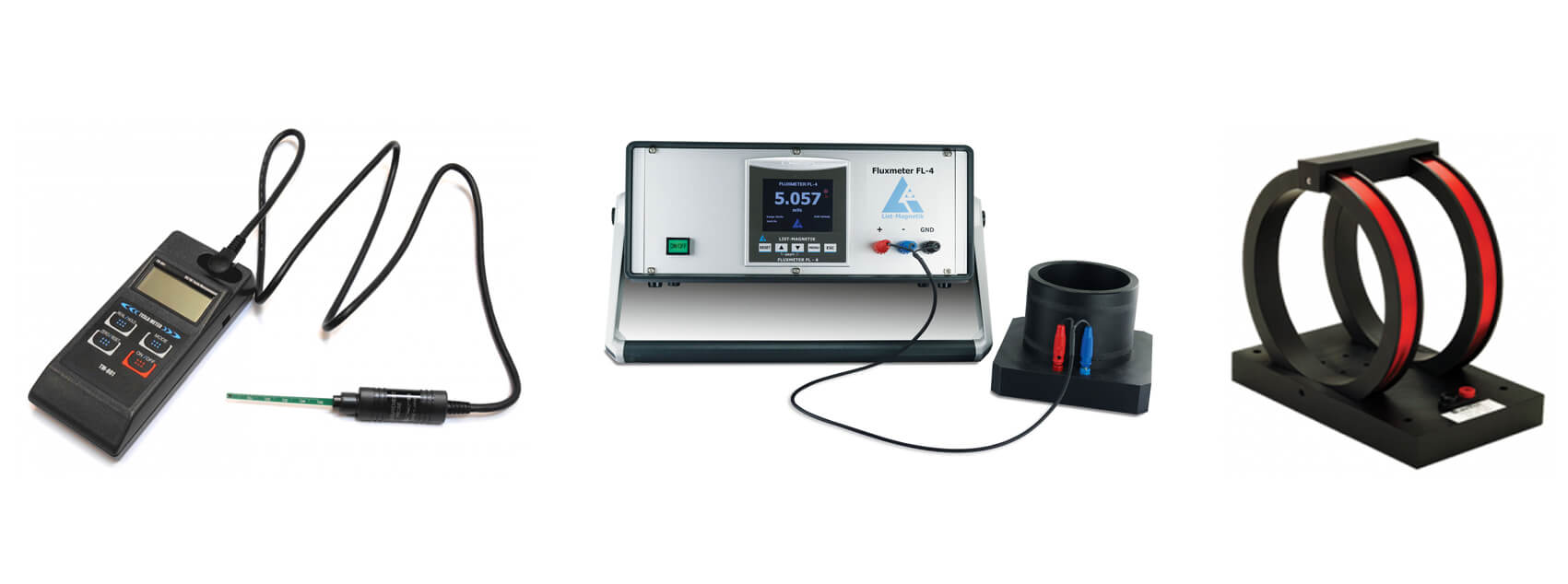## Influence Factors of Surface Gauss

As a non-uniform magnetic field, different positions on the magnet’s surface have different gauss values. Non multi-pole magnet with simple shape is generally taking geometric center’s gauss value as acceptance criteria. Gauss value of magnetizing surface’s geometric center can be approximately calculated via Biot-Savart law:

#### Calculation Formula of Cylinder Shape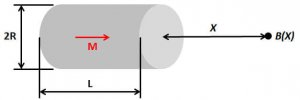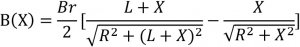Where X is the air gap between testing point and magnet’s surface.

#### Calculation Formula of Block Shape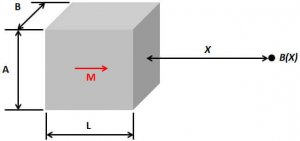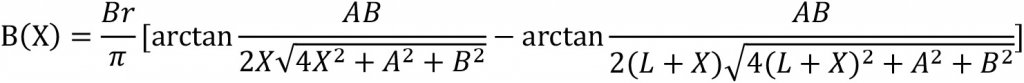Where X is the air gap between testing point and magnet’s surface.

#### Calculation Formula of Ring Shape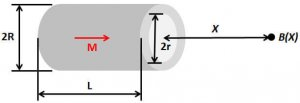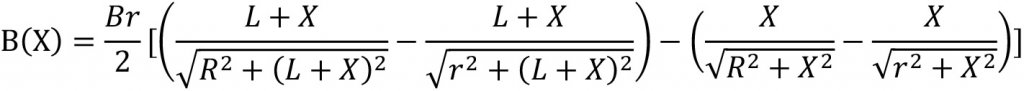Where X is the air gap between testing point and magnet’s surface.

It can be concluded from above calculation formulas that gauss values are directly affected by grade, dimension, and testing point.

Gaussmeters are comprised of hall probe and instrument module. Gauss value decrease with increasing air gap, thus encapsulation of hall component, or a neglected air gap, has the critical role on the accuracy of gauss value. That means gauss value very likely different even when different Gaussmeters or probes are testing the same point of one magnet. But in fact, measurement repeatability of gauss value is very low even testing point is the geometric center. It must be noted that surface gauss cannot represent the overall magnetic performance of the magnet compared with magnetic flux or magnetic moment.

## 1 comment

1. i want to know exact derivation process of ‘Calculation Formula of Ring Shape’.